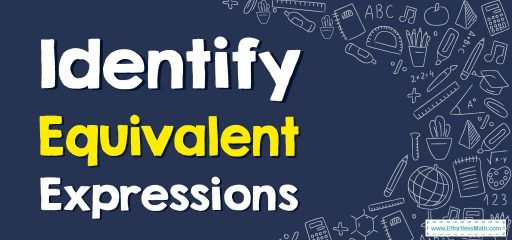# How to Identify Equivalent Expressions?

In this article, the focus is on teaching you how to recognize when two expressions are equivalent, regardless of their form or appearance. By understanding how to identify equivalent expressions, you can simplify and manipulate mathematical expressions more effectively.“Equivalent” refers to the property of being equal in value or meaning. When applied to mathematical expressions, it means that two expressions represent the same numerical value for all possible inputs.

## A step-by-step guide toidentifying equivalent expressions

Here is a step-by-step guide to identifying equivalent expressions:

1. Review the properties of arithmetic operations: Understanding the properties of operations like associativity, commutativity, and distributivity is essential to identifying equivalent expressions.
2. Use the order of operations: Ensure that you evaluate both expressions using the same order of operations (PEMDAS) to compare their values.
3. Simplify each expression: Simplify each expression as much as possible before comparing them.
4. Replace variables: If the expressions contain variables, substitute the same value for the variable in both expressions to compare their numerical values.
5. Check for a common factor: Look for a common factor that can be pulled out from both expressions to make them easier to compare.
6. Apply rules of algebra: Use the rules of algebra, such as the distributive property and the commutative property, to rearrange and simplify both expressions.
7. Compare the expressions: Finally, compare both expressions by seeing if they are equal in value for all inputs. If they are, they are equivalent expressions.

By following these steps, you can systematically approach the task of identifying equivalent expressions and ensure that you arrive at the correct answer.

### Identify Equivalent Expressions – Example 1

Determine the equivalent expression.
$$9w$$$$-$$$$4w$$$$+$$$$5$$
Solution:
To form the standard form, combine like terms.
$$9w$$$$-$$$$4w$$$$=$$$$5w$$
$$5w$$$$+$$$$5$$

### Identify Equivalent Expressions – Example 2

Determine the equivalent expression.
$$29d$$$$-$$$$11d$$$$+$$$$7$$$$-$$$$10d$$
Solution:
To form the standard form, combine like terms.
$$29\:?\:−11\:?−10\:?=9\:?$$
$$9d$$$$+$$$$7$$

## Exercises forIdentify Equivalent Expressions

### Determine the equivalent expression.

1. $$\color{blue}{xy\: (9z+7)}$$
2. $$\color{blue}{\:x\left(9\:+\:3x\right)}$$
3. $$\color{blue}{6y\:+\:2y\:+\:3y\:+\:4y}$$
1. $$\color{blue}{9xyz\:+\:7xy}$$
2. $$\color{blue}{9x\:+\:3x^2}$$
3. $$\color{blue}{15y}$$

### What people say about "How to Identify Equivalent Expressions? - Effortless Math: We Help Students Learn to LOVE Mathematics"?

No one replied yet.

X
30% OFF

Limited time only!

Save Over 30%

SAVE $5 It was$16.99 now it is \$11.99